Next: Implementation Up: Kantorovitch theorem Previous: Kantorovitch theorem   Contents

Mathematical background

Let a system ofequations inunknowns: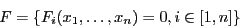each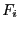being at least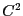. Let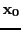be a point and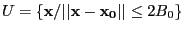, the norm being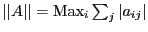. Assume thatis such that:
1. the Jacobian matrix of the system has an inverse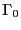atsuch that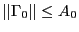2.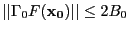3.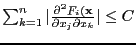for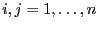and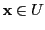4. the constants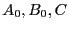satisfy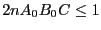Then there is an unique solution of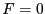in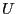and Newton method used withas estimate of the solution will converge toward this solution . An interesting use of Kantorovitch theorem can be found in section 2.5.

Jean-Pierre Merlet 2012-12-20